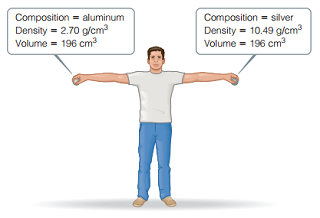# Problem: You may want to reference (Pages 15 - 16) Section 1.4 while completing this problem.Consider the two spheres shown here, one made of silver and the other of aluminum.Does the act of lifting the sphere off the ground increase the potential energy of the aluminum sphere by a larger amount, a smaller amount, or the same amount as the silver sphere?

###### FREE Expert Solution

We are asked if the act of lifting the sphere off the ground increases the potential energy of the aluminum sphere by a larger amount, a smaller amount, or the same amount as the silver sphere.

93% (491 ratings)###### Problem Details

You may want to reference (Pages 15 - 16) Section 1.4 while completing this problem.

Consider the two spheres shown here, one made of silver and the other of aluminum.Does the act of lifting the sphere off the ground increase the potential energy of the aluminum sphere by a larger amount, a smaller amount, or the same amount as the silver sphere?